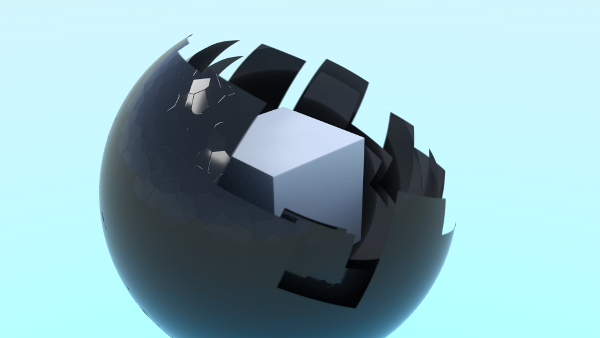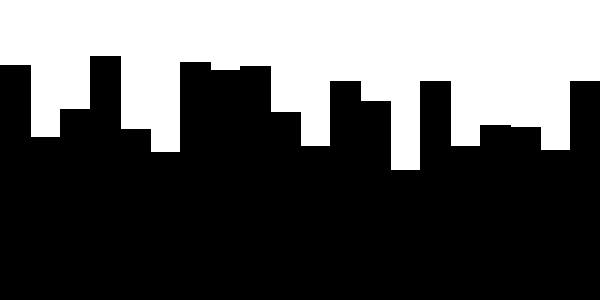## growing texture

For this animation I used an image sequence to switch between a transparent and an opaque material in cycles.

To generate the image texture I wrote a processing sketch that animates black stripes growing from bottom to top at various speeds. (see below for the sourcecode of the processing sketch)this is the processing sketch I used to generated the image sequence for the animated texture

``````float[] speed = new float;
float[] h = new float;
float w;
float f;
void setup() {
size(600,300);
fill(0);
for ( int i=0; i<speed.length; i++) {
speed[i]= random( 0.5, 1);
h[i]=0;
}
w = width/speed.length;
f = float(height)/250.0/0.5;
}

void draw() {
background(255);
fill(0);
for ( int i=0; i<speed.length; i++) {
if (h[i] < height) h[i]+= speed[i]*f;
rect( i*w, height-h[i], w, h[i] );
}
if ( frameCount <= 250 ) {
saveFrame( "grow-####.png");
}
}
``````

and here is an example what the generated texture looks like